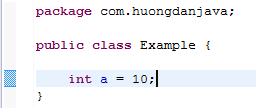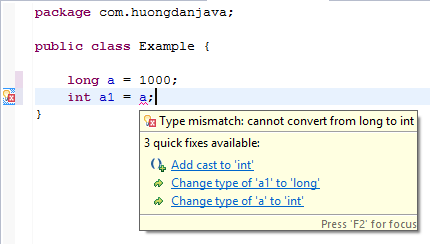# Assignment operator in Java

In Java, we have the following assignment operators:

• =
• +=
• -=
• *=
• /=

– The assignment operator “=” is used most often, which is used to initialize the variable and assign a new value to it.

Example:You cannot use this assignment operator to assign a boolean value to a variable of type char, byte, short, int, long or float, or double and vice versa. If you try to do that then the compile error will occur.You cannot assign a variable whose data type has a larger range value to a variable whose value type has a smaller range value.

For example, we cannot assign a variable of type long to a variable of type int:That’s because the range value of the long type is greater than int.

The remaining operators include “+ =”, “- =”, “* =”, “/ =” are abbreviations for summation, subtraction, multiplication and division with the assignment operator.

• “+=”: first plus then assign.
• “-=”: the first is the subtraction followed by the assignment.
• “*=”: first multiply then assign.
• “/=”: first divide then assign.

For example, we have the following calculation:

which means that

Or

which means that

Similarly to divide and multiply.